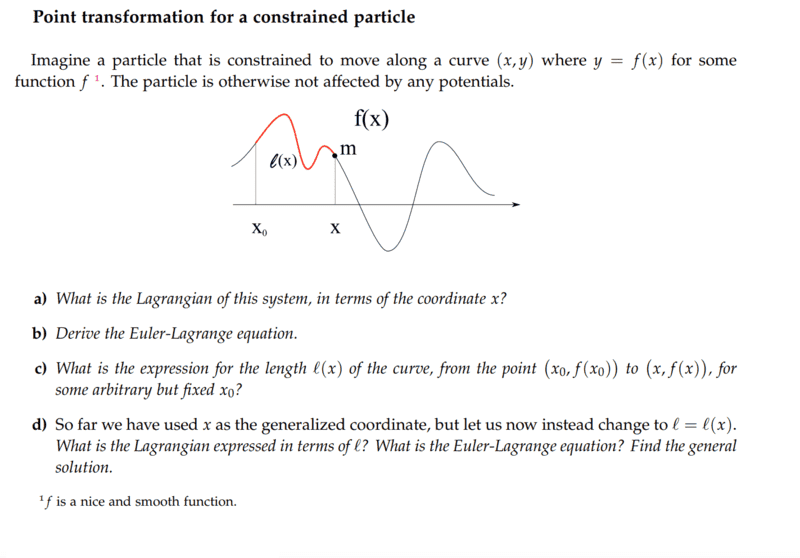# Point transformation for a constrained particle

• Lambda96
In summary, pasmith is not very familiar with the Lagrangian formalism and he is having difficulty solving task 1a. He has now proceeded by assuming that the potential energy is zero and has found the kinetic energy. He is Unsure about task b as he is not sure if the equation depends on x. He has then found the Euler-Lagrange equation.f

#### Lambda96

Homework Statement
Lagrangian mechanics for a mass particle
Relevant Equations
none
Hi,

unfortunately, I'm not that fit concerning the Lagrangian formalism, so I'm not sure if I solved the problem 1a correctly.I have now proceeded as follows

the Lagrangian is

$$L=T-U$$

Since there are no constraining or other forces acting on the point mass, I assume that the potential energy is 0 and thus the system has only kinetic energy, i.e.

$$T=\frac{1}{2}m*(\dot{x}^2+\dot{y}^2)$$

I would now represent the entire equation in the x coordinate only, so.

$$x=x$$
$$y=f(x)$$

Insert into the Lagrangian

$$T=\frac{1}{2}m*(\dot{x}^2+\dot{f}^2(x))$$

Thus, I would be done with task 1a, or did I do something wrong?

That is correct, except that if $y = f(x)$ then $\dot y = f'(x)\dot x$, so $$T = \frac12 m (1 + f'(x)^2)\dot x^2$$.

•PhDeezNutz
Thank you pasmith for your help, I had completely forgotten the chain rule.

Concerning task b, the Euler-Lagrange equation is as follows

$$\frac{\partial L}{\partial x}-\frac{d}{dt}\frac{\partial L}{\partial \dot{x}}=0$$

For $$\frac{\partial L}{\partial x}$$

I am not quite sure since f(x) depends on x, would the following then apply

$$\frac{\partial L}{\partial x}=m f''(x)$$

and

$$\frac{\partial L}{\partial \dot{x}}=m\dot{x}+m f'(x)^2\dot{x}$$

$$\frac{d}{dt}m\dot{x}+m f'(x)^2\dot{x}=m\ddot{x}+m f''(x)^2\ddot{x}\dot{x}$$

Then the Euler-Lagrange equation is

$$m f''(x)-m\ddot{x}-m f''(x)^2\ddot{x}\dot{x}=0$$

Your lagrangian is $L = \frac12 m g(x)\dot x^2$ where $g(x) = 1 + f'(x)^2$. Hence $$\begin{split} \frac{d}{dt}\left( \frac{\partial L}{\partial \dot x}\right) - \frac{\partial L}{\partial x} &= \frac{d}{dt}(mg(x)\dot x) - \tfrac12 m g'(x) \dot x^2 \\ &= mg(x) \ddot x + mg'(x) \dot x^2 - \tfrac12 m g'(x) \dot x^2 \\ &= mg(x) \ddot x + \tfrac12 m g'(x) \dot x^2. \end{split}$$ So what is $g'(x)$ using the chain rule?

$$\frac{\partial L}{\partial \dot{x}}=m\dot{x}+m f'(x)^2\dot{x}$$

$$\frac{d}{dt}m\dot{x}+m f'(x)^2\dot{x}=m\ddot{x}+m f''(x)^2\ddot{x}\dot{x}$$
Don't forget the product rule.

$$\frac{\partial L}{\partial \dot{x}}=m\dot{x}+m f'(x)^2\dot{x}$$

$$\frac{d}{dt}m\dot{x}+m f'(x)^2\dot{x}=m\ddot{x}+m f''(x)^2\ddot{x}\dot{x}$$
This is not good!

I suggest you take ##f(x) = \sin x## or something like that and check your differentiation against a definite function.

I don't want to be rude, but you are missing trivial points about elementary calculus, as chain rule and product rule.

May i ask, have you take those class? Don't try to skip steps in your learning process.

Thanks to all for the help, especially pasmithI have now formed the derivative of ##g(x)=1+f'(x)^2## as follows.

The outer derivative is ##2f'(x)##

and the inner derivative would be ##f''(x)##

Thus, the derivative of ##g'(x)## would be ##g'(x)=2f'(x)f''(x)##

The Euler-Lagrange equation is ##m\ddot{x}+mf'(x)^2\ddot{x}+mf'(x)f''(x)\dot{x}^2##

Concerning task part c and d